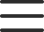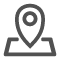# java中如何构建Random产生随机数？

Random类似Scanner，也是Java提供好的API，内部提供了产生随机数的功能 API后续课程详细讲解，本节课中可以简单理解为Java已经写好的代码 。Random产生随机数的步骤如下：

1. 导入包

import java.util.Random;

2. 创建对象

Random r = new Random();

3. 产生随机数

int num = r.nextInt(10);

```import  java.util.Random;
public  class RandomDemo  {
public  static void  main(String[]  args)  {
//创建对象
Random  r =  new  Random();
//用循环获取10个随机数
for(int  i=0;  i<10;  i++)  {
//获取随机数
int  number  =  r.nextInt(10);
System.out.println("number:"  +  number);
}
//需求：获取一个1-100之间的随机数
int  x  =  r.nextInt(100)  +  1;
System.out.println(x);
}
}```

Random案例：猜数字

A. 如果猜的数字比真实数字大，提示你猜的数据大了
B. 如果猜的数字比真实数字小，提示你猜的数据小了
C. 如果猜的数字与真实数字相等，提示恭喜你猜中了

```import java.util.Random;
import java.util.Scanner;
public class RandomTest {
public static void main(String[] args) {
//要完成猜数字的游戏，首先需要有一个要猜的数字，使用随机数生成该数字，范围1到100
Random r = new Random();
int number = r.nextInt(100) + 1;

while(true) {
//使用程序实现猜数字，每次均要输入猜测的数字值，需要使用键盘录入实现
Scanner sc = new Scanner(System.in);
System.out.println("请输入你要猜的数字：");
int guessNumber = sc.nextInt();
//比较输入的数字和系统产生的数据，需要使用分支语句。
//这里使用if..else..if..格式，根据不同情况进行猜测结果显示
if(guessNumber > number) {
System.out.println("你猜的数字" + guessNumber + "大了");
} else if(guessNumber < number) { System.out.println("你猜的数字" + guessNumber + "小了");
} else { System.out.println("恭喜你猜中了");
break;
}
}

}
}```

Python的标准模块：sys、os、random和time

random随机数用法

random模块介绍